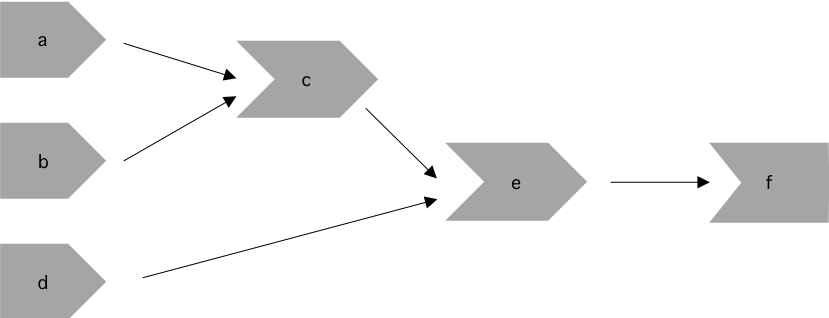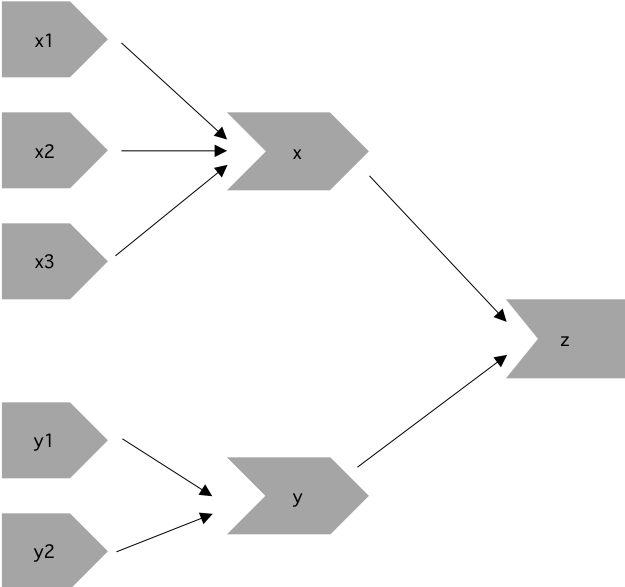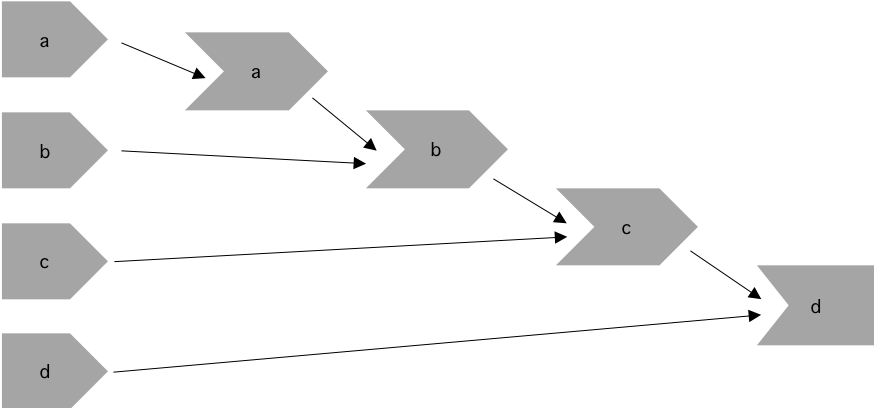# Chapter 4 Basic Reactivity

### Exercise 4.3.6.1

Draw the reactive graph for the following server functions:

``````server1 <- function(input, output, session) {
c <- reactive(input\$a + input\$b)
e <- reactive(c() + input\$d)
output\$f <- renderText(e())
}

server2 <- function(input, output, session) {
x <- reactive(input\$x1 + input\$x2 + input\$x3)
y <- reactive(input\$y1 + input\$y2)
output\$z <- renderText(x() / y())
}

server3 <- function(input, output, session) {
d <- reactive(c() ^ input\$d)
a <- reactive(input\$a * 10)
c <- reactive(b() / input\$c)
b <- reactive(a() + input\$b)
}``````

#### Solution. Solution

To create the reactive graph we need to consider the inputs, reactive expressions, and outputs of the app.

For `server1` we have the following objects:

• inputs: `input\$a`, `input\$b`, and `input\$d`
• reactives: `c()` and `e()`
• outputs: `output\$f`

Inputs `input\$a` and `input\$b` are used to create `c()`, which is combined with `input\$d` to create `e()`. The output depends only on `e()`.For `server2` we have the following objects:

• inputs: `input\$y1`, `input\$y2`, `input\$x1`, `input\$x2`, `input\$x3`
• reactives: `y()` and `x()`
• outputs: `output\$z`

Inputs `input\$y1` and `input\$y2` are needed to create the reactive `y()`. In addition, inputs `input\$x1`, `input\$x2`, and `input\$x3` are required to create the reactive `x()`. The output depends on both `x()` and `y()`.For `server3` we have the following objects:

• inputs: `input\$a`, `input\$b`, `input\$c`, `input\$d`
• reactives: `a()`, `b()`, `c()`, `d()`

As we can see below, `a()` relies on `input\$a`, `b()` relies on both `a()` and `input\$b`, and `c()` relies on both `b()` and `input\$c`. The final output depends on both `c()` and `input\$d`.### Exercise 4.3.6.2

Why will this code fail?

``````var <- reactive(df[input\$var])
range <- reactive(range(var(), na.rm = TRUE))``````

Why is `var()` a bad name for a reactive?

#### Solution. Solution

This code doesn’t work because we called our reactive `range`, so when we call the `range` function we’re actually calling our new reactive. If we change the name of the reactive from `range` to `col_range` then the code will work. Similarly, `var()` is not a good name for a reactive because it’s already a function to compute the variance of `x`! `?cor::var`

``````library(shiny)

df <- mtcars

ui <- fluidPage(
selectInput("var", NULL, choices = colnames(df)),
verbatimTextOutput("debug")
)

server <- function(input, output, session) {
col_var <- reactive( df[input\$var] )
col_range <- reactive({ range(col_var(), na.rm = TRUE ) })
output\$debug <- renderPrint({ col_range() })

}

shinyApp(ui = ui, server = server)``````

### Exercise 4.4.6.1

Use reactive expressions to reduce the duplicated code in the following simple apps.

#### Solution. Solution

Unclear about the apps mentioned in the exercise.